# Algebra - math word problems

1. A rectangular patioA rectangular patio measures 20 ft by 30 ft. By adding x feet to the width and x feet to the length, the area is doubled. Find the new dimensions of the patio.
2. The crime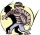The crime rate of a certain city is increasing by exactly 7% each year. If there were 600 crimes in the year 1990 and the crime rate remains constant each year, determine the approximate number of crimes in the year 2025.
3. Sides of triangle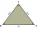Triangle circumference with two identical sides is 117cm. The third side measures 44cm. How many cms do you measure one of the same sides?
4. Find the sumFind the sum of all natural numbers from 1 and 100, which are divisible by 2 or 5
5. Triangle 42Triangle BCA. Angles A=119° B=(3y+14) C=4y. What is measure of triangle BCA=?
6. Equation 20In given equation: 8/9-4/5=2/9+x, find x
7. MG=7x-15,MG=7x-15, FG=33, x=? Point M is the midpoint of FG. Find unknown x.
8. Completing squareSolve the quadratic equation: m2=4m+20 using completing the square method
9. Find the 7Find the number that is smaller than 5 5/12 by as much as 2 2/13 is smaller than 6 1/6
10. Two numbers 7The sum of two consecutive even numbers is 30. Find the numbers.
11. Evening shiftWhile working the evening shift, Officer K took 8 hours to complete a task at his work station and Officer M took 10 hours to complete the same task at his work station. How many hours would it take Officer K and Officer M to complete the same task working
12. Simple equation 6Solve equation with one variable: X/2+X/3+X/4=X+4
13. Toys 3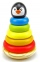Tiffany's toyshop received a shipment of 360 toys. The first day 12 were sold the second day 19 were sold and on the third day, 26 was sold. How many days will the toyshop run out of toys?
14. Eq with reciprocalSolve given equation with reciprocal member: a-6/a+10=4/8
15. Substitution method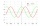Solve goniometric equation: sin4 θ - 1/cos2 θ=cos2 θ - 2
16. Expression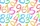If A=2 B=3 evaluate expression A(B+A) and multiply it by A
17. WhichWhich decimals when subtracted equal 3.89: a - b = 3.89
18. The sizeThe size of a Trapezium are 3/4×cm, ×cm 2(×+1)cm and 3(×+2)cm long respectively if it's perimeter is 60cm, calculate the length of each side.
19. A report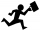Maricris can type a committee report in 5 hours. Jane helped Maricris and together they finished the report in 3 hours. How long would it take Jane to complete the report she had worked alone?
20. Lateral surface areaThe ratio of the area of the base of the rotary cone to its lateral surface area is 3: 5. Calculate the surface and volume of the cone, if its height v = 4 cm.

Do you have an interesting mathematical word problem that you can't solve it? Enter it, and we can try to solve it.

To this e-mail address, we will reply solution; solved examples are also published here. Please enter the e-mail correctly and check whether you don't have a full mailbox.

Please do not submit problems from current active competitions such as Mathematical Olympiad, correspondence seminars etc...